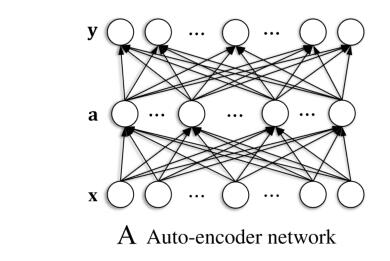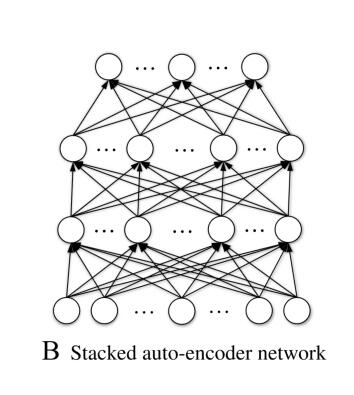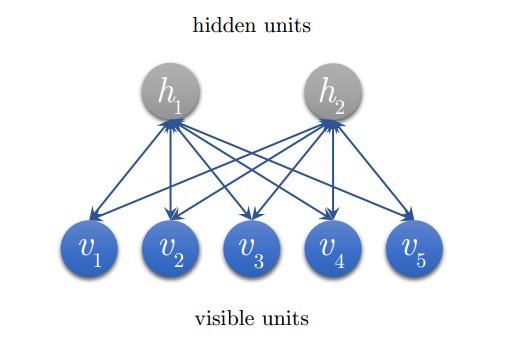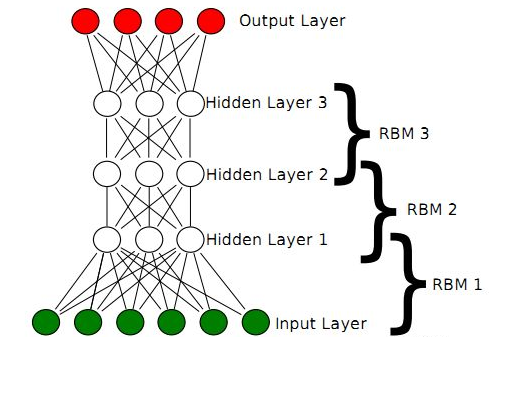# 深度学习：自编码器、深度信念网络和深度玻尔兹曼机

## 一、自编码器

### 1. 自编码器### 2. 稀疏自编码器

J ( W , b ) = E ( x , y ) + γ ∑ j D H K L ( ρ ∣ ∣ ρ j ^ ) J(W,b)=E(x,y)+\gamma\sum_j^{D_H}KL(\rho||\hat{\rho_j})

K L ( ρ ∣ ∣ ρ j ^ ) = ρ log ⁡ ρ ρ j ^ + ( 1 − ρ ) l o g 1 − ρ 1 − ρ j ^ KL(\rho||\hat{\rho_j})=\rho\log\frac{\rho}{\hat{\rho_j}}+(1-\rho)log\frac{1-\rho}{1-\hat{\rho_j}}

### 3. 堆栈自编码器## 二、深度信念网络和深度玻尔兹曼机

### 1. 受限玻尔兹曼机### 2. 深度信念网络### 3. 深度玻尔兹曼机07-131588
07-132万+
03-26689
09-203万+
07-092万+
06-261万+
11-251万+
12-135万+
01-071万+